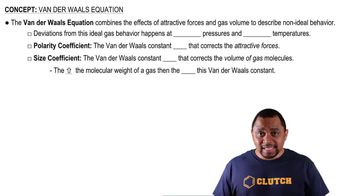Start typing, then use the up and down arrows to select an option from the list.
9:27 minutes
Problem 148
Textbook Question

# The Rankine temperature scale used in engineering is to the Fahrenheit scale as the Kelvin scale is to the Celsius scale. That is, 1 Rankine degree is the same size as 1 Fahrenheit degree, and 0 °R = absolute zero. (b) What is the value of the gas constant R on the Rankine scale in 1L ~ atm2>1°R ~ mol2? (c) Use the van der Waals equation to determine the pressure inside a 400.0-mL vessel that contains 2.50 mol of CH4 at a temperature of 525 °R. For CH4, a = 2.253 1L2 ~ atm2>mol2 and b = 0.04278 L>mol.Verified Solution
This video solution was recommended by our tutors as helpful for the problem above.
95views

### Watch next

Master Van der Waals Equation with a bite sized video explanation from Jules Bruno

Start learning01:4003:1903:55